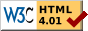## Dokumentation zu: test_bit(E)``` SYNOPSIS int test_bit(string str, int n) DESCRIPTION Return 0 or 1 of bit n was set in string str. Each character contains 6 bits. So you can store a value between 0 and 63 in one character (2^6=64). Starting character is the blank " " which has the value 0. The first character in the string is the one with the lowest bits (0-5). EXAMPLES test_bit("_",5); Returns 1 because "_" stands for the number 63 and therefore the 6th bit is set. test_bit(" ",3); Returns 0 because " " stands for 0 and no bit is set. SEE ALSO set_bit(E), clear_bit(E), last_bit(E), next_bit(E), count_bits(E), and_bits(E), or_bits(E), xor_bits(E), invert_bits(E), copy_bits(E) ``` Die Seite ist auch in Deutsch vorhanden.

Start » Magierhandbuch » Docu » Efun » Test_bit Letzte Generierung: 25.04.2021, 01:58mud@wl.mud.de